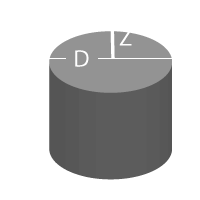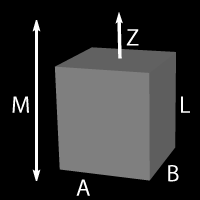# Magnetic Field Strength Calculators

The calculators on this page are appropriate only for "Squre Loop", also known as "Straight Line", magnetic materials such as neodymium-iron-boron, ferrites and samarium cobalt magnets. They are not for use with alnico materials.

### Field Strength of a Cylindrical Magnet

Z = Distance tested from magnet
L = Length of magnet
D = Diameter of magnet
Br = Residual Gauss of magnet

 Z: in. L: in. D: in. Br: gaussB(z) = Gauss

### Field Strength of a Rectangular Magnet

Z = Distance tested from magnet
L = Length of magnet
A = Height of magnet
B = Width of magnet
Br = Residual Gauss of magnet

 Z: in. A: in. B: in. L: in. Br: gaussB(z) = Gauss